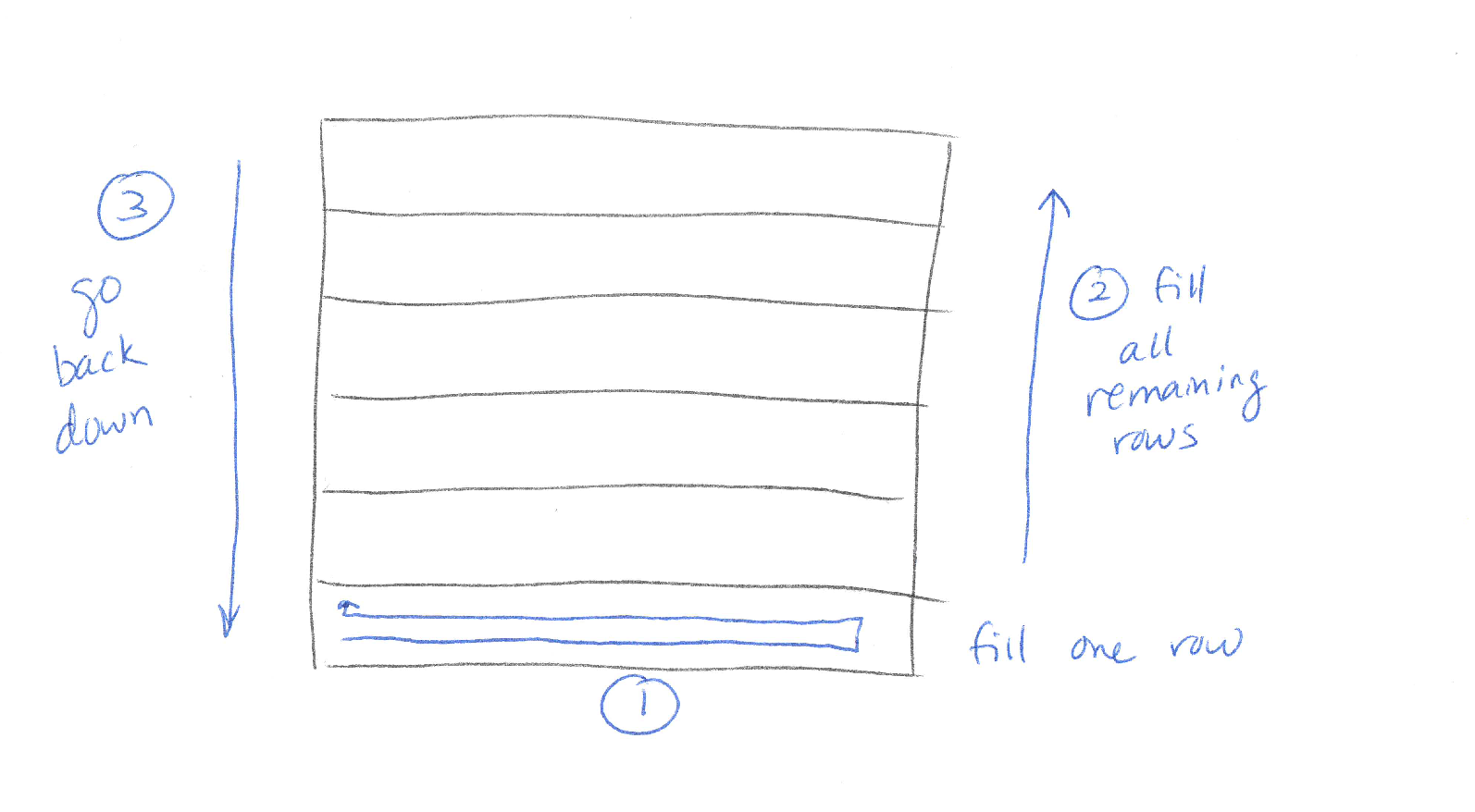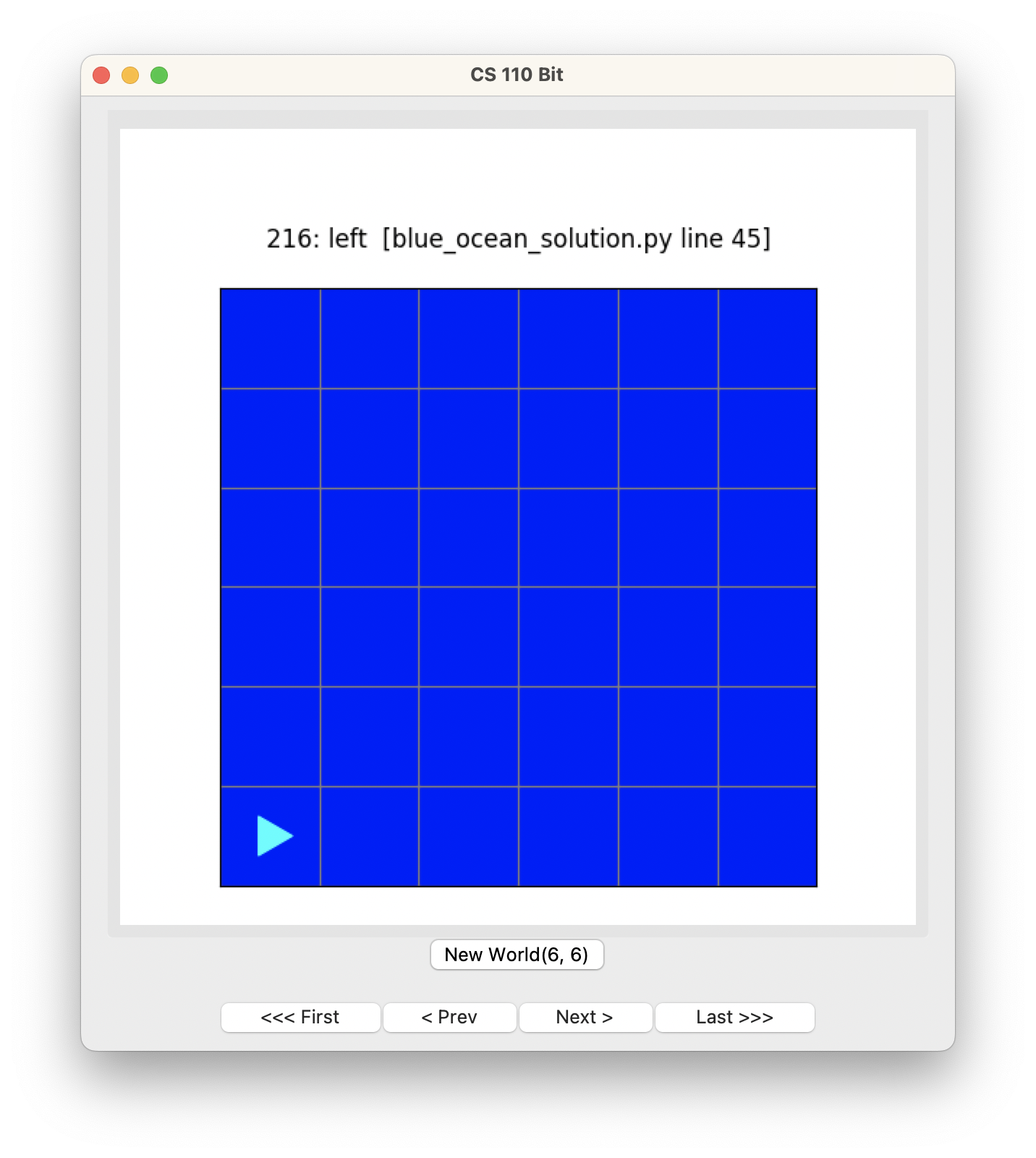Computer Science

# Blue ocean

This problem will help you practice using while loops and decomposing problems.

Bit starts in this world:Your job is to have Bit fill the world with a blue ocean, and end up back where they started:## Starter code

In your `bit` folder, create a file called `blue_ocean.py`. Copy and paste this code into the file:

``````from byubit import Bit

@Bit.empty_world(6, 6)
def run(bit):
# Write code here
pass

if __name__ == '__main__':
run(Bit.new_bit)
``````

## Planning

Before you write any code, plan out your solution with pencil and paper. Find a friend and draw out how you think you would solve this problem.What did you draw? Maybe you decided to fill one row, go up, and fill the next row going in the opposite direction:• Step 1: Fill a pair of rows
• Step 2: Fill the rest of the rows
• Step 3: Go back to the start

Or maybe you decided to fill one row, then go back to the beginning of the row:• Step 1: Fill a single row
• Step 2: Fill the rest of the rows
• Step 3: Go back to the start

Let’s talk about these approaches for a minute:

(1) First, we want to use a loop to fill in all the rows. Don’t just copy and paste code 3 times (if you are doing pairs) or 6 times (if you are doing one row at a time).

(2) Second, to make this loop work, it is a good idea to end the first step facing up. This will allow you to use a while loop to fill in the rest of the rows, because you can keep fill rows as long as the front is clear.

(3) You can technically use either approach, filling one or two rows at a time. But filling pairs of rows only works if the number of rows is even! If we had 5 or 7 rows, the solution would break.

For this reason, we are going to use the sketch where we fill one row at a time.

## Decomposing the problem

We start at the highest or most abstract level and write functions. For example:

``````def run(bit):
""" Fill an ocean by doing one row at a time. """
fill_one_row(bit)
while bit.front_clear():
# move up and turn right
bit.move()
bit.right()
# fill one row
fill_one_row(bit)

go_back_to_start(bit)``````

This solution has Bit fill one row and then end facing up. Then we keep moving up one row and filling rows as long as there is space to do this. Finally, we move back to the start.

Remember, PyCharm will put squiggly red lines underneath the functions you haven’t defined yet. Hover over these and select `Create function` in blue to create empty functions for each of them.

## Filling one row

How would you fill one row and then move back to the start of the row?In this case, filling a row consists of multiple parts — painting the row, and then going back. In cases like this, the best thing to do is to repeat the decomposition step and make two new functions:

``````def fill_one_row(bit):
""" Fill one row """
# go forward, painting blue
paint_row(bit)
# turn around and go back
turn_around(bit)``````

Then you can fill these in:

``````def paint_row(bit):
""" Paint an entire row blue """
bit.paint('blue')
while bit.front_clear():
bit.move()
bit.paint('blue')``````

This function paints the entire row blue, starting with the first square.

``````def turn_around(bit):
""" Turn around and go back to the start of the row.
End facing up.
"""
# turn around
bit.right()
bit.right()
# move back
while bit.front_clear():
bit.move()

# face up
bit.right()``````

This function turns around, moves all the way back, and then faces up.

Use small functions that do one thing well

If you put all of that code into one, big, `fill_one_row()` function, your code will still work, it will just not be as beautiful. It is easier to write and maintain code that has been decomposed into small functions.

Now let’s stop and run this code to be sure it works:Hey, we filled all the rows! This is because our main `run()` function re-uses our `fill_one_row()` function:

``````def run(bit):
""" Fill an ocean by doing one row at a time. """
fill_one_row(bit)
while bit.front_clear():
# move up and turn right
bit.move()
bit.right()
# fill one row
fill_one_row(bit)

go_back_to_start(bit)``````

The only thing we have left is to write `go_back_to_start()`.

## A debugging interlude

You will probably not write your code corretly the first time. In that case, you will need to debug your code. Try removing `bit.right()` in the `run()` function. Run the code and see what it does. Use the buttons to see why this happens.

## Go back to the start

OK, back to coding. How would you go back to the start?Here is one way to do this:

``````def go_back_to_start(bit):
""" Go back to the start. """
# turn around
bit.right()
bit.right()
# move back
while bit.front_clear():
bit.move()

# turn to face right (the way we started)
bit.left()``````

We need to turn around, move back, and then get Bit facing the right way. Run the code:We did it!